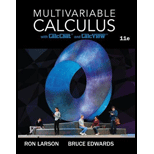# Wronskian The Wronskian of two differentiable functions f and g , denoted by W (f, g), is defined as the function given W ( f , g ) = | f g f ' g ' | . The function f and g are linearly independent when there exists at least one value of x for which W ( f , g ) ≠ 0 . In exercise 71-74, use the Wronskian to verify that the two functions are linearly independent. y 1 = e a x y 2 = e b x , a ≠ b### Multivariable Calculus

11th Edition
Ron Larson + 1 other
Publisher: Cengage Learning
ISBN: 9781337275378

#### Solutions

Chapter
Section### Multivariable Calculus

11th Edition
Ron Larson + 1 other
Publisher: Cengage Learning
ISBN: 9781337275378
Chapter 16.2, Problem 71E
Textbook Problem
16 views

## Wronskian The Wronskian of two differentiable functions f and g, denoted by W (f, g), is defined as the function given W ( f , g ) = | f g f ' g ' | .The function f and g are linearly independent when there exists at least one value of x for which W ( f , g ) ≠ 0 . In exercise 71-74, use the Wronskian to verify that the two functions are linearly independent. y 1 = e a x y 2 = e b x ,     a ≠ b

To determine

To verify:

The two function y1=eaxandy2=ebx,ab are linearly independent by using Wronskian Method.

### Explanation of Solution

Given information:

The given function are y1=eaxandy2=ebx,ab.

Concept Used:

The Wronskian of two differentiable functions f and g, denoted by W (f, g), is defined as the function given by the determinant W(f,g)=|fgf'g'|.

Proof:

First, find the derivative of the y1andy2 with respect to x, we get

y1'=aeaxandy2'=bebx

Now, we have to find the determinant W(y1,y2)=|y1y2y1'y2'|

### Still sussing out bartleby?

Check out a sample textbook solution.

See a sample solution

#### The Solution to Your Study Problems

Bartleby provides explanations to thousands of textbook problems written by our experts, many with advanced degrees!

Get Started

Find more solutions based on key concepts
Bounced-Check Charges Overdraft fees have become an important part of a banks total fee income. The following t...

Applied Calculus for the Managerial, Life, and Social Sciences: A Brief Approach

In Exercises 516, evaluate the given quantity. log22

Finite Mathematics and Applied Calculus (MindTap Course List)

Evaluate the integral. 46. 0/41cos4d

Single Variable Calculus

(y)2 + 6xy 2y + 7x = 0 is a differential equation of order ______. a) 1 b) 2 c) 3 d) 4

Study Guide for Stewart's Single Variable Calculus: Early Transcendentals, 8th

Change each common fraction to a decimal: 1115

Elementary Technical Mathematics

Evaluate the definite integrals in Problems 1-32. 27.

Mathematical Applications for the Management, Life, and Social Sciences

What is the value of (X + 1) for the following scores: 0, 1, 4, 2? a. 8 b. 9 c. 11 d. 16

Essentials of Statistics for The Behavioral Sciences (MindTap Course List)

Series Discount This is a continuation of Exercise 11. Sometimes manufacturers give more than one discount inst...

Functions and Change: A Modeling Approach to College Algebra (MindTap Course List)

Consider the Costello Music Company problem in exercise 48. The quarterly sales data follow.

Modern Business Statistics with Microsoft Office Excel (with XLSTAT Education Edition Printed Access Card) (MindTap Course List)

Solve Problem 21 assuming that pure water is pumped into the tank. 21. A tank contains 200 liters of fluid in w...

A First Course in Differential Equations with Modeling Applications (MindTap Course List)